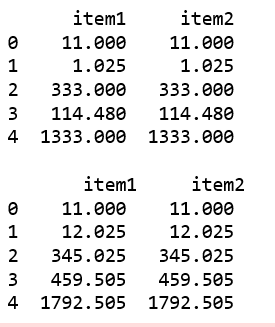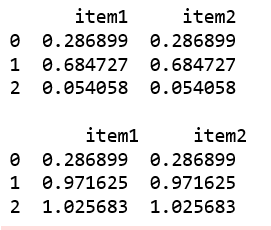Open in App
Not now

# Python | Pandas Panel.cumsum()

• Last Updated : 01 Jan, 2019

In Pandas, Panel is a very important container for three-dimensional data. The names for the 3 axes are intended to give some semantic meaning to describing operations involving panel data and, in particular, econometric analysis of panel data.

`Panel.cumsum()` function is used to returns a DataFrame or Series of the same size containing the cumulative sum.

Syntax: Panel.cumsum(axis=None, skipna=True, *args, **kwargs)

Parameters:
axis : The index or the name of the axis. 0 is equivalent to None or ‘index’.
skipna : Exclude NA/null values. If an entire row/column is NA, the result will be NA.

Returns: Cumsum of DataFrame or Panel

Code #1:

 `# importing pandas module ``import` `pandas as pd ``import` `numpy as np`` ` `df1 ``=` `pd.DataFrame({``'a'``: [``'Geeks'``, ``'For'``, ``'geeks'``, ``'for'``, ``'real'``], ``                    ``'b'``: [``11``, ``1.025``, ``333``, ``114.48``, ``1333``]})``                     ` `data ``=` `{``'item1'``:df1, ``'item2'``:df1}`` ` `# creating Panel ``panel ``=` `pd.Panel.from_dict(data, orient ``=``'minor'``)`` ` `print``(panel[``'b'``])`` ` `print``(``"\n"``, panel[``'b'``].cumsum(axis ``=` `0``))`

Output:Code #2:

 `# importing pandas module ``import` `pandas as pd ``import` `numpy as np`` ` `df1 ``=` `pd.DataFrame({``'a'``: [``'Geeks'``, ``'For'``, ``'geeks'``], ``                    ``'b'``: np.random.randn(``3``)})``                     ` `data ``=` `{``'item1'``:df1, ``'item2'``:df1}`` ` `# creating Panel ``panel ``=` `pd.Panel.from_dict(data, orient ``=``'minor'``)`` ` `print``(panel[``'b'``])`` ` ` ` `print``(``"\n"``, panel[``'b'``].cumsum(axis ``=` `0``))`

Output:My Personal Notes arrow_drop_up